Next: Hamilton's Principle Up: Hamiltonian Dynamics Previous: Conditional Variation

Multi-Function Variation

Suppose that we wish to maximize or minimize the functional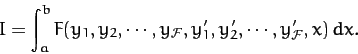(707)

Here, the integrandis now a functional of the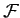independent functions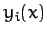, for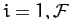. A fairly straightforward extension of the analysis in Section 10.2 yieldsseparate Euler-Lagrange equations,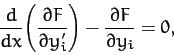(708)

for, which determine thefunctions. Ifdoes not explicitly depend on the function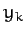then the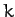th Euler-Lagrange equation simplifies to(709)

Likewise, ifdoes not explicitly depend onthen allEuler-Lagrange equations simplify to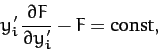(710)

for.

Richard Fitzpatrick 2011-03-31